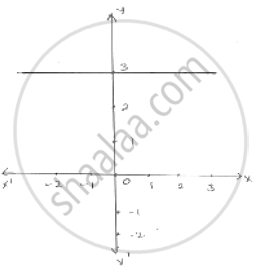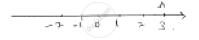# Give the Geometric Representation of the Following Equation (A) on the Number Line (B) on the Cartesian Plane : Y = 3 - Mathematics

Give the geometric representation of the following equation
(a) on the number line   (b) on the Cartesian plane :   y = 3

#### Solutiony  = 3 .
Point A represents 3on number line
On Cartesian plane, equation represents all points on x  - axis  for which  y = 3

Concept: Equations of Lines Parallel to the X-axis and Y-axis
Is there an error in this question or solution?

#### APPEARS IN

RD Sharma Mathematics for Class 9
Chapter 7 Linear Equations in Two Variables
Exercise 7.4 | Q 1.3

Share